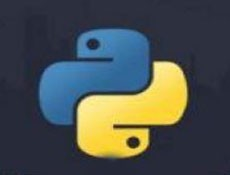# python里input怎么解释• silencement   2019-10-10 15:21:512243python中input函数有类似c中的scanf函数的功能。

Python2中input使用如下：

```>>>x = input("x:")
x: 3
>>>y = input("y:" )
y: 4
>>> print x*y
12```

```>>> x = input("x:")
x:3
>>> y = input("y:")
y:4
>>> print (x*y)
Traceback (most recent call last):
File "<stdin>", line 1, in <module>
TypeError: can't multiply sequence by non-int of type 'str'
>>>```

Python3以后的版本中，raw_input和input合体了，取消了raw_input，并用input代替，所以说现在版本的input接受的是字符串，可以如下处理：

```>>> x = int(input("x:"))
x:3
>>> y = int(input("y:"))
y:4
>>> print (x*y)
12```